Name:    Chapter 8 Test

Multiple Choice
Identify the choice that best completes the statement or answers the question.

Write the word sentence as an inequality.

1.

A number x divided by 6 is at most 47.
 a.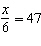c.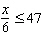b.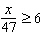d.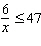Graph the inequality on a number line.

2.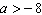a.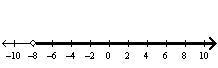c.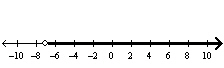b.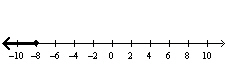d.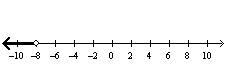3.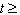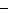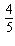a.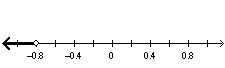c.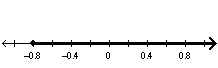b.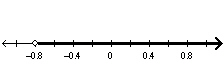d.Solve the inequality. Graph the solution.

4.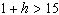a.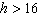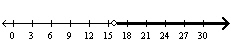c.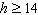;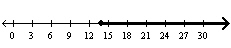b.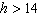;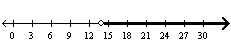d.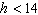;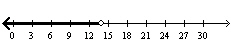5.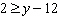a.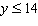;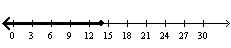c.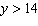;b.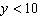;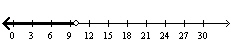d.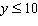;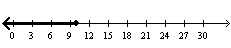6.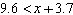a.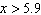;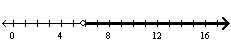c.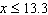;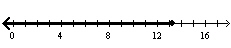b.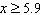;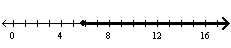d.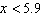;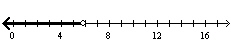7.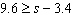a.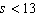;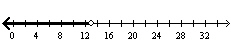c.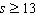;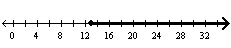b.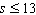;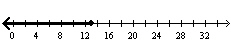d.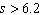;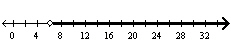8.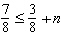a.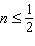;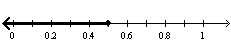c.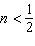;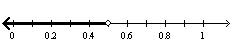b.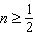;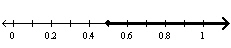d.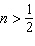;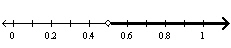9.

Your carry-on bag can weigh at most 40 pounds. Your bag weighs in at 25 pounds. Write and solve an inequality to represent how much more weight you can add to the bag and still meet the requirement.
 a.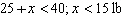c.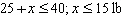b.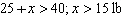d.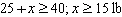Write the word sentence as an inequality. Then solve the inequality.

10.

255 is no less than 15 times a number w.
 a.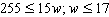c.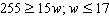b.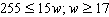d.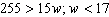Solve the inequality. Graph the solution.

11.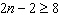a.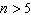;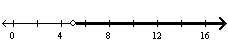c.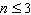;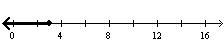b.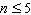;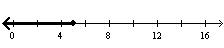d.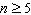;12.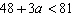a.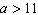;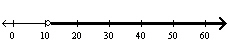c.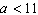;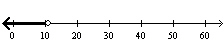b.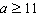;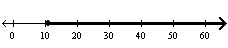d.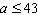;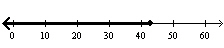13.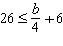a.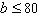;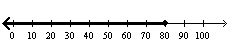c.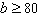;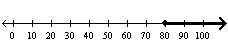b.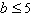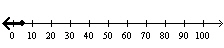d.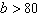;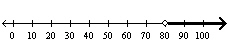1.

Are the graphs of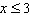and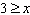the same or different? Explain.

Write an inequality and a word sentence that represent the graph.

2.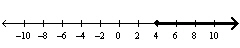Other

1.

Extended Response  A drop tower in an amusement park, holds a maximum of 18 people per ride.
a.   Write and solve an inequality to find the least number of rides needed for 5,400 people.
b.   The amusement park is open from noon until 10 P.M. each day. Do you think it is possible for 5,400 people to ride this drop tower in one day? Explain.

2.

Extended Response  A killer whale has eaten 65 pounds of fish today. It needs to eat at least 130 pounds of fish each day.
a.   A bucket holds 15 pounds of fish. Write and solve an inequality to represent how many more buckets of fish the whale needs to eat.
b.   Should the whale be given four or five more buckets of fish? Explain.